# Scalar QCD renormalization

• idmena
In summary, the student is trying to figure out what is wrong with their calculations after adding the contributions from the diagrams shown. They are not able to factorize their result and think the problem is due to a missing 1/6 S term.f

#### idmena

Hello all, I hope you can give me a hand with a QFT homework I'm working on. We are to compute the beta equation of a Non-abelian SU(N) theory with: Complex scalars (massless), bosons, ghosts. My question is referring to the Boson self-energy scalar loop correction.

1. Homework Statement

We have the boson self energy correction involving a scalar loop.
This loop is formed of 2 3-vertex of Boson-scalar-scalar: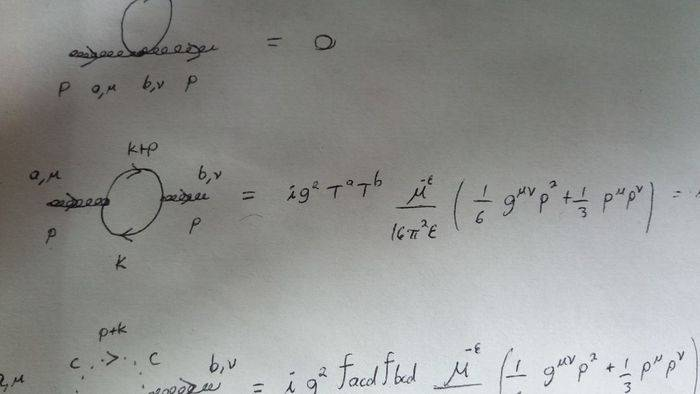## Homework Equations

The Feynman rules I derived for this diagrams are: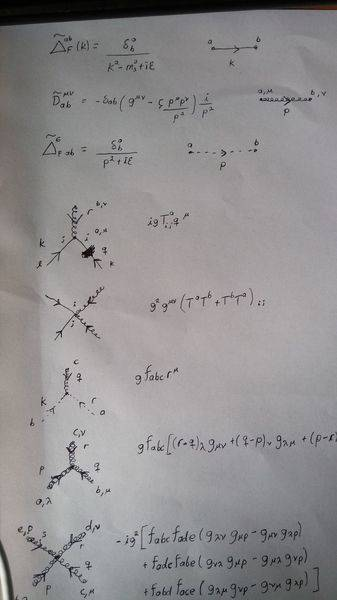Where solid lines are scalars and dashed lines are ghosts.

## The Attempt at a Solution

This is what I get: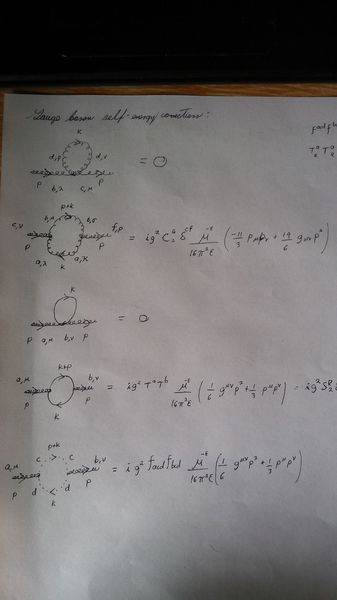(We are setting the scalars to be massless). I know the boson loop and the ghost loop are corrrect as I checked them on a book (I'm using Bailin & Love).
The reason of my confusion is, when I add them all I get, besides some factors: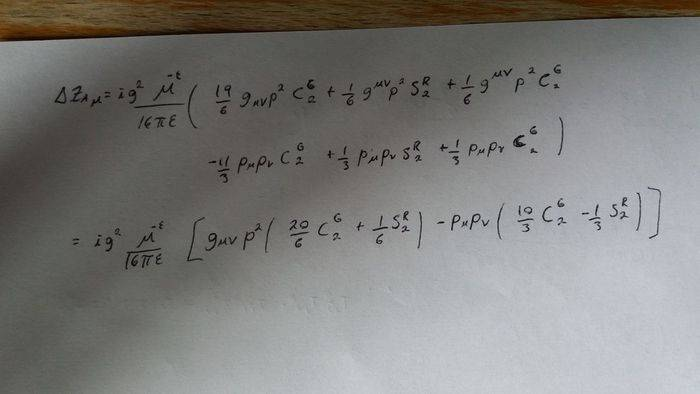But on the lecture our teacher told us we should get: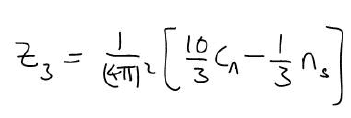I have a sign and a factor on 2 wrong, and It's coming from the scalar loop diagram.

As we can see from the feynman rules, the 3-vertex for boson-scalar is the same as the one for boson-ghost, except for a factor. Doesn't these mean that both self-energy corrections should give me the same answer, except for such factor? And, therefore, if I know the ghost loop is correct, then I also know the scalar loop should have the same form (given the scalars are massless, as mentioned previously). But then, I am missing the (-) sign and the factor of two.

Can you help me with this please? Can someone confirm the Feynman rule I got for boson-scalar-scalar is correct? If so, where might the problem be?

Thank you very much
Regards

Hello all, I hope you can give me a hand with a QFT homework I'm working on. We are to compute the beta equation of a Non-abelian SU(N) theory with: Complex scalars (massless), bosons, ghosts. My question is referring to the Boson self-energy scalar loop correction.

Hi, I am trying to make sure I understand the question correctly. Where exactly do you think you are off by a factor of 2 and a minus sign? What do you get for your ##Z_3##? (I can see what I would get using your expression but I want to make sure we are on the same wavelength in terms of notation)

Hi, I am trying to make sure I understand the question correctly. Where exactly do you think you are off by a factor of 2 and a minus sign? What do you get for your ##Z_3##? (I can see what I would get using your expression but I want to make sure we are on the same wavelength in terms of notation)

Adding the contributions from the diagrams shown, I get what is shown in the first line of the last pic: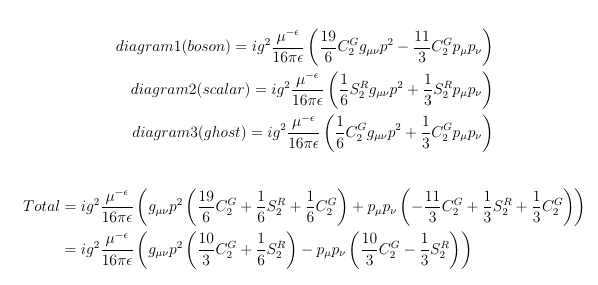The counter term in my lagrangean should look like:
(propagator) * (something)

So, at this point I would like to have something like: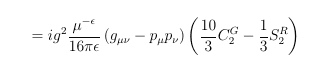Alas, I cannot do this becuase there is a 1/6 S that does not let me factorize my result like this. That term comes from the scalar loop, that is why I think that is the prpblem.

By the way, I should've mentioned before that I am working in Feynman gauge, so I should only have the propagator counter-term, not a gauge fixing counter-term.